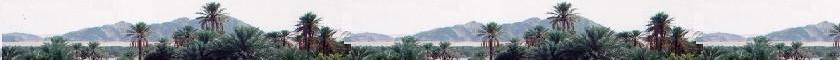Quantum Mechanics

Schrodinger equation

Quantum Mechanics

Propagators : Pg

Quantum Simple Harmonic
Oscillator QSHO

Quantum Mechanics
Simulation With GNU Octave

# Hermite polynomials

### 1. Hermite polynomials

```
In Physics, Hermite polynomials are used give determine the
eigenstates of the quantum harmonic oscillator. They are the
set of sequences:

The Hermite differential equation is:

y"(x) - 2xy'(x) + 2ny(x) = 0

The solution of this non-linear second-order ordinary differential
equation is the set of Hermite polynomials.

Hn = (- 1)n exp{x2} dn(exp{- x2})/dxn.
Hn is the polynomial of degree n.

Here some first sequences:

H0(x) = 1
H1(x) = 2x
H2(x) = 4x2 - 2
H3(x) = 8x3 - 12x
H4(x) = 16x4 - 48x2 + 12
H5(x) = 32x5-160x3 + 120x

The Hermite polynomial are orthogonal with the
weight w(x) = exp{- x2}. Thus:
∫ Hn(x) Hm(x) exp{- x2} dx = δ(n,m) [x: from - ∞ to ∞]

Recursion relations

Hn+1(x) = 2xHn(x)- H'n(x)
H'n(x) = 2nHn - 1(x)
Hn+1(x) = 2xHn(x) - 2nHn- 1(x)

```

### 2. Quantum Simple Harmonic Oscillator (QSHO)

```2.1. Schr�dinger�s Equation for QSHO
The Schr�dinger�s equation for QSHO is:

- ħ 2/2m Ψ"(x) + (1/2) k x2 Ψ(x) - E Ψ(x) = 0
or:

Ψ"(x) + [ (2m/ħ2)E - (mk/ħ2) x2]Ψ(x) = 0

Where 2πħ is the Planck constant,
m is the mass of the particle, k is the spring constant and
E is the energy of the particle.

Let's write:
x = z/α then:
Ψ'(x) = α Ψ'(z)
Ψ"(x) = α2 Ψ"(z)

Therefore
α2 Ψ"(z) + [ (2m/ħ2)E - (mk/ħ2) (z/α)2]Ψ(x) = 0

Dividing by α2 , yields:

Ψ"(z) + [ (2m/ħ2  α2 )E - (mk/ħ2) (z2/α)4]Ψ(x) = 0

Let's define: km/ħ2α4 = 1, so

α4 = mk/ħ2

We have then:
Ψ"(z) + [ (2m/ħ2 α2 )E - z2]Ψ(x) = 0

Now, let's define:

Ψn (z) = exp{- z2/2} Hn(z)

Therefore:
Ψ'n (z) = - z Ψn (z) + exp{- z2/2} H'n(z)
Ψ"n (z) = - Ψn - zΨ'n (z) + exp{- z2/2} H"n(z)
- z exp{- z2/2} H'n(z) =

Ψ"(z)n (z) = - Ψn - z [- z Ψn (z) +
exp{- z2/2} H'n(z)] + exp{- z2/2} H"n(z)
- z exp{- z2/2} H'n(z)
= - Ψn + z2Ψn (z) - z exp{- z2/2} H'n(z) +
exp{- z2/2} H"n(z) - z exp{- z2/2} H'n(z)
= (z2 - 1)Ψn (z) - 2xexp{- z2/2} H'n(z) + exp{- z2/2} H"n(z)

The Schrodinger's equation becomes:
z2Ψ - Ψn (z) - 2 z exp{- z2/2} H'n(z) +
exp{- z2/2} H"n(z) + [ (2mE/ħ2α2) - z2]Ψ(z) = 0

Or:
- 2 z  H'n(z) +  H"n(z) + [ (2mE/ħ2α2) - 1]H(z) = 0

Rearranging:
H"n(z) - 2 z  H'n(z) + [(2mE/ħ2α2) - 1]H(z) = 0

then the Schrodinger's equation becomes the Hermite
equation with:

2mE/ħ2α2) - 1 = 2n, so

E = (ħ2α2/2m) (2n + 1)

We have α2/2m = (k/m)1/2/2ħ, then
E = (ħ2(k/m)1/2/2ħ) (2n + 1)
= (ħ(k/m)1/2) (n + 1/2)

Let's write:
ω2 = k/m

Therefore:
E  = ħω (n + 1/2)

E  = ħω (n + 1/2)

The wave function becomes:

Ψn (z) =  Ψn (z) = exp{- z2/2} Hn(z)
is the solution of the schrodinger equation, with
z = α x
α4 = mk/ħ2.
E = (ħ2α2/2m) (2n + 1)

```
##### 2.2. Normalization of the function Ψn (z)
```The hermite polynomials are orthogoal as follows:

∫ exp{- z2} Hn(z) Hm dz = (2n π1/2 n!)δ(n,m) [from _∞ to ∞]

Then:
∫ |Ψn (z)|dz = 1, so
∫ N2 exp{- z2} H2n(z) = 1  [from _∞ to ∞]
Then
N = [n!2n π1/2]- 1/2

Therefore:
Ψn (z) = [n! 2n π1/2]- 1/2 exp{- z2/2} Hn(z)

or:
Ψn (x) = [n! 2n π1/2]- 1/2 exp{- (αx)2/2} Hn(x)

The results are:

Ψn (x) = [n! 2n π1/2]- 1/2 exp{- (αx)2/2} Hn(x)
E = (ħ2α2/2m) (2n + 1) = ħω (n + 1/2)
α4 = mk/ħ2  ω2 = k/m
The energy is not null even at the ground
state where n = 0.
The transition energy between states is ħω.

```

 chimie labs | Physics and Measurements | Probability & Statistics | Combinatorics - Probability | Chimie | Optics | contact |